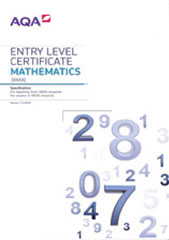# 3.3 Component 3: ratio

This content aims to develop the student’s understanding of equality and basic fractions.

## Procedures for making and recording assessments

Class work assessed by the teacher in response to the outcomes in the table, or assessed by an externally set assignment.

## Outcomes to be accredited

In successfully completing this component, students will have demonstrated the ability to:

### Entry 1

Outcomes

Notes/examples

GCSE reference

1.1 Understand equality

Use and understand the = sign

2 + 5 = 5 + ?

N1

1.2 Identify or show one half of a quantity up to 20

Given a picture of children,

What fraction of the children are boys?

N12, R6

1.3 Work out half of an even number up to 20

N12, R6

### Entry 2

Outcomes

Notes/examples

GCSE reference

2.1 Identify or show one third or one quarter of a quantity up to 24

Shade one third or one quarter of a shape

Given a picture of children,

What fraction of the children are boys?

N12, R6

2.2 Work out one third or one quarter of a number up to 24

Without remainder

N12, R6

2.3 Count in fractions of one half or one third or one quarter

Give the next number in the pattern:

2, ,

2.4 Work out amounts two, three or four times the size of a given amount

Key words are double, twice (as ..), three times (as..), four times (as..)’

One loaf of bread has 22 slices. How many slices are there in 4 loaves ?

R6

2.5 Recognise the equivalence of and

### Entry 3

Outcomes

Notes/examples

GCSE reference

3.1 Identify or show unit fractions up to one tenth of a quantity up to 100

Shade a given unit fraction of a specified grid

Given a picture of children,

What fraction of the children are boys?

N12, R6

3.2 Work out unit fractions to one tenth of a number up to 100

Without remainder

N12, R6

3.3 Identify or show any number of thirds, quarters, fifths or tenths of a quantity

Shade a fraction of a shape

Given a picture of children,

What fraction of the children are boys?

N12

3.4 Work out any number of thirds, quarters, fifths or tenths of an amount

Without remainder

N12

3.5 Recognise and identify equivalent fractions

N3

3.6 Add and subtract fractions with the same denominator within one whole

N8

3.7 Work out amounts 5, 8 or 10 times the size of a given amount

R2